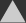# Total Solution for Structural OptimizationOPTISHAPE-TS

## tech spec

optimization function Non-parametric Shape Optimization Topology Optimization / Die Drawing Topology Optimization Bead Optimization Linear Static Analysis, Geometrical non-linear Analysis, Eigen Analysis, Buckling Eigen Analysis Solver, Steady State Heat Conduction and Static Coupled Analysis, Frequency Response Analysis, Sound-Structure Interaction Analysis Solver input Nastran Bulk ANSYS CDB output Nastran Bulk Nastran OUTPUT2 Nastran DVGRID I-DEAS Universal FEMAP Neutral ANSYS RST STL TS Studio OPTISHAPE-TS Navigator Graph Viewer 'TS Graph' Analysis Scheduling Software 'TS Scheduler'
objective/constraint function Volume (Total/Design Region) Mass (Total/Design Region) Total Moment of inertia Surface Area (Total/Design Region) Shape Variation Location of the center of gravity Compliance Maximum Mises stress Maximum principal stress Maximum principal strain Maximum principal shearing stress Displacement Absolute value of principal strain Negative Eigen Value Displacement of eigen mode Squared error of eigen mode Maximum thermal stress ( Mises stress ) Thermal displacement Negative value of buckling load factor Maximum Mises stress multiple Subcase(prerelease function) Amplitude of displacement on frequency analysis Amplitude of Velocity on frequency analysis Amplitude of Acceleration on frequency analysis Sound pressure (linear) Sound pressure (dB) Overlap Volume with the Target Domain Deficit Volume from the Target Domain Deviation Volume from the Target Domain Uniform Cross Section Restriction for die drawing Constraint not to going through a plane Constraint not to going through a cylindrical wall Shape of cross section Constraint of shape variation in normal direction of the shell elements Design area defined by arbitrary shape Restriction of minimum thickness Keeping uniform thickness Keeping rib shape
objective/constraint function Volume (Total/Design Region) Mass (Total/Design Region) Compliance Displacement Eigen Value Uniform Section Symmetric Symmetric Section 1/4 Symmetric 1/4 Symmetric Section Cylinder Axisymmetric
objective/constraint function Compliance Gray Scale Area Roughness of Bead Eigenvalue * Option.
OS Windows : Windows 10 [64bit] Linux (solver only)* : Red Hat Enterprise Linux (RHEL) 5, 6, 7 SUSE Linux Enterprise Server (SLES) 11, 12 « Quinr License System (based on FLEXNET Publisher) » Windows : Windows 10 Windows Server 2012 Linux* : Red Hat Enterprise Linux 6, 7 [64bit] SUSE Linux Enterprise Server 11, 12 [64bit] * Option.

_product